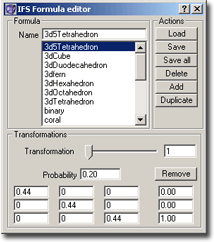### Formula Editor for IFSFig.: Formula Editor for IFS fractals

This chapter assumes, that you know about the theoretical basis of iterated function systems. If not, then please read chapter IFS Theory.

Well, the purpose is to define a system of affine transformations (matrix plus vector), where each affine transformation has assigned a probability, which determines how often this transformation should be used. ChaosPro now is able to calculate the attractor of such an IFS.

The list box lists all currently known IFS formulas. At program startup ChaosPro scans the formula directories for formula files having the extension *.ifs. All those files are scanned, all IFS formulas found in them are automatically loaded. Above this list box you can change the name of the formula.

Right beneath there are six buttons, which offer the following actions:

• Load an IFS formula file: After pressing that button, you are prompted for a formula file containing IFS formulas to be loaded. ChaosPro is able to read all FractInt IFS formula files (for example Fractint.ifs).
• Save the IFS formula into a formula file. This function lets you save the currently selected IFS formula into a formula file. If you specify an existing formula file, then ChaosPro will scan that file, searching for an entry with the same name as the formula to be saved. If an entry is found, ChaosPro asks you, if you want to replace that entry with the currently selected formula, or if you simply want to add your formula to the end of that file.
• Save All: This function saves all IFS formulas into a formula file.
• Delete the currently selected IFS formula.
• Add a new IFS formula.
• Clone the currently selected IFS formula.

Below the list box you can specify the transformations of the currently selected IFS formula. At first you will see the transformation slider. This slider lets you choose the transformation. If you move the slider, you will notice, that the last transformation for each formula is “empty”: This allows you to add transformations. If you want to add a transformation, then simply choose the last one and type in some values. As soon as you press return, the transformation gets created. After that the formula has one more transformation, the new one is the last but one, and the last transformation again is empty…

If you want to delete a transformation, simply press the button ‘Remove’.

The transformation itself consists of a probability, a 3×3 matrix and a vector.

Each field can not only contain a number (as in FractInt). ChaosPro allows you to specify any arithmetic expression using the built in formula editor. The arithmetic expression can contain any parameter mentioned in chapter Parameters and any function listed in chapter Functions. As soon as you leave the entry field (i.e. it loses the keyboard focus) , the arithmetic expression is examined (parsed). If you mispelled something, an error will appear. See chapter Error Messages for possible errors and what causes them.

This feature allows you to specify p1, p2, p3 or p4 in any field. These parameters refer to parameter 1 to 4 in IFS Parameter Window 1. And these parameters are changed during animation (because they belong to the IFS fractal itself). So by building a reference from an IFS formula to a fractal using such a parameter you can calculate an animation, where the probability of a transformation continuously changes.